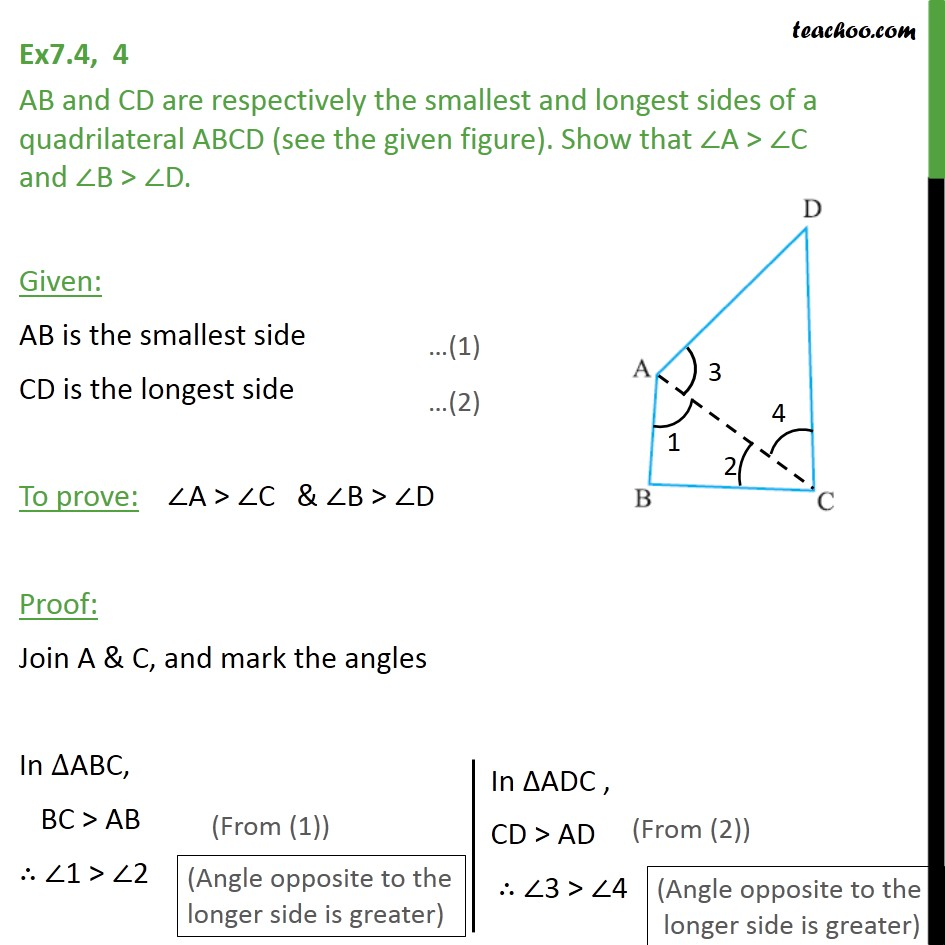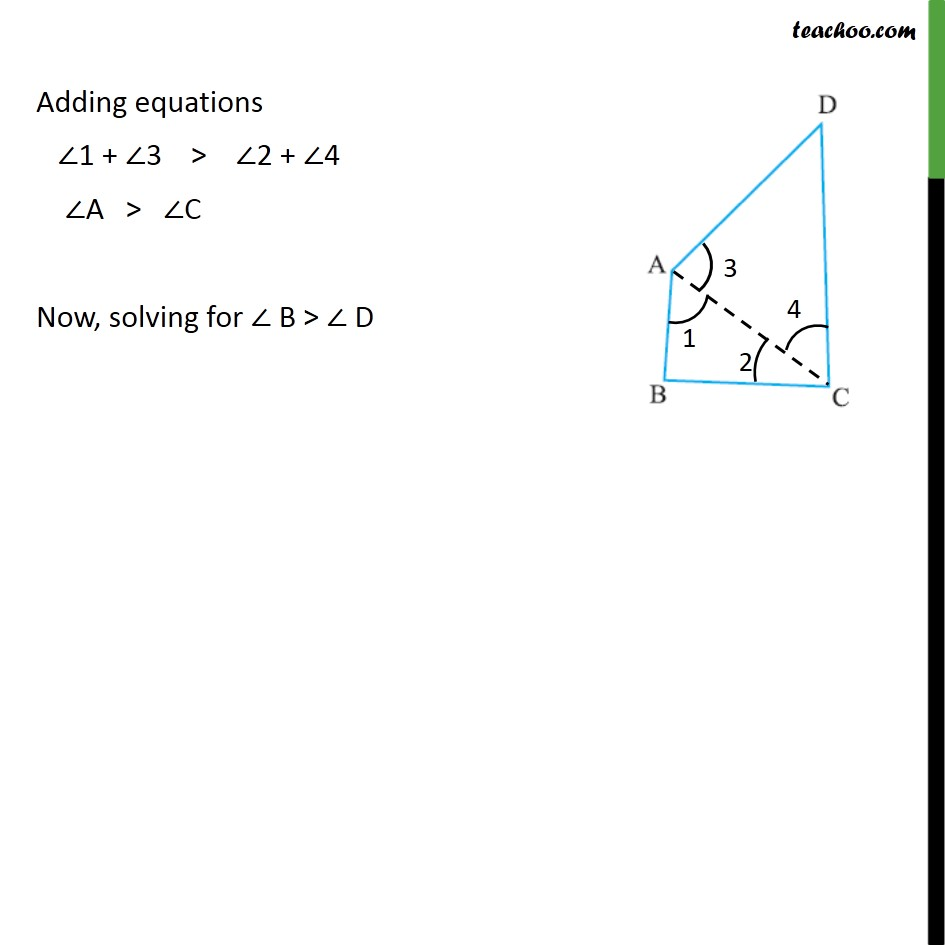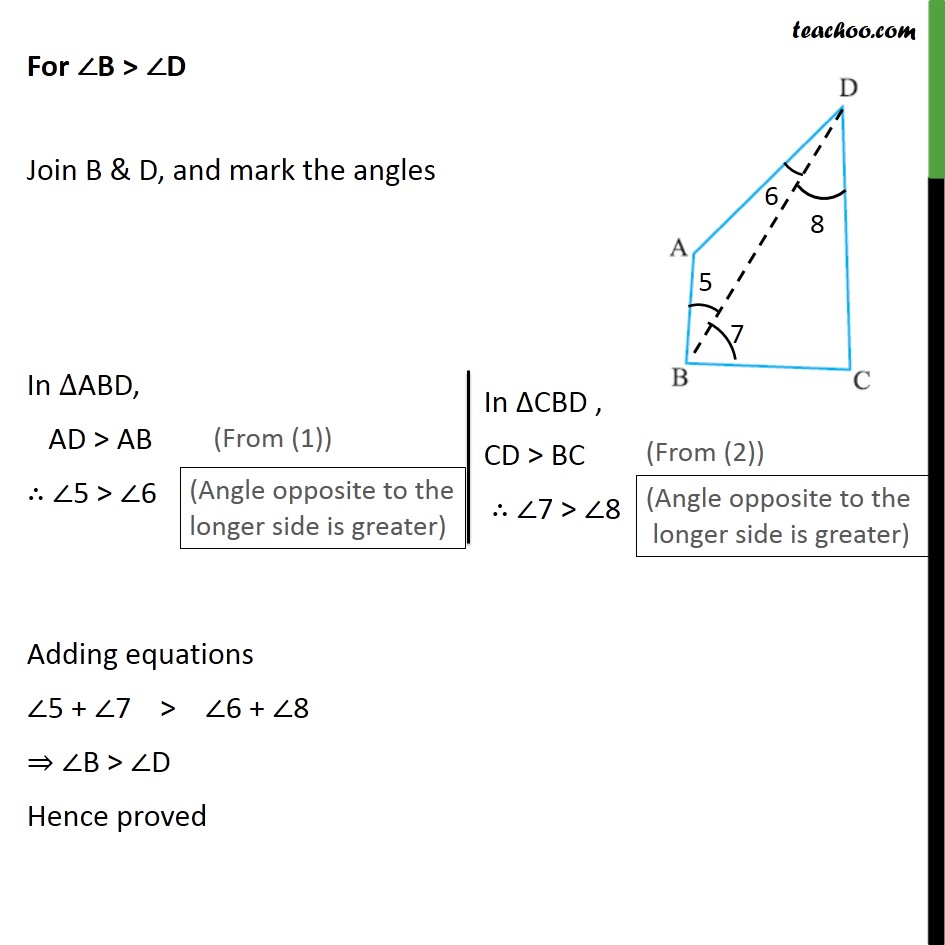1. Chapter 7 Class 9 Triangles
2. Serial order wise
3. Ex 7.4

Transcript

Ex7.4, 4 AB and CD are respectively the smallest and longest sides of a quadrilateral ABCD (see the given figure). Show that ∠A > ∠C and ∠B > ∠D. Given: AB is the smallest side CD is the longest side To prove: ∠A > ∠C & ∠B > ∠D Proof: Join A & C, and mark the angles In ∆ABC, BC > AB ∴ ∠1 > ∠2 Adding equations ∠1 + ∠3 > ∠2 + ∠4 ∠A > ∠C Now, solving for ∠ B > ∠ D For ∠B > ∠D Join B & D, and mark the angles In ∆ABD, AD > AB ∴ ∠5 > ∠6 Adding equations ∠5 + ∠7 > ∠6 + ∠8 ⇒ ∠B > ∠D Hence proved

Ex 7.4

Chapter 7 Class 9 Triangles
Serial order wise